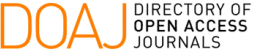### On the Stability of Volterra Difference Equations of Convolution Type

Higidio Portillo Oquendo, Jose Renato Ramos Barbosa, Patricia Sánez Pacheco

#### Abstract

In \cite{Elaydi-10}, S.\ Elaydi obtained a characterization of the stability of
the null solution of the Volterra difference equation
\beqae
\eeqae
by localizing the roots of its characteristic equation
\beqae
1-\sum_{n=1}^{\infty}a_nz^n=0\textrm{.}
\eeqae
The assumption that $(a_n)\in\ell^1$ was the single hypothesis considered for
the validity of that characterization, which is an insufficient condition if the
ratio $R$ of convergence of the power series of the previous equation equals
one. In fact, when $R=1$, this characterization conflicts with a result obtained
by Erd\"os et al in \cite{Erdos}. Here, we analyze the $R=1$ case and show that
some parts of that characterization still hold. Furthermore, studies on
stability for the $R<1$ case are presented. Finally, we state some new results
related to stability via finite approximation.

#### Keywords

difference equation, stability, convolution.

#### Full Text:

PDF

DOI: https://doi.org/10.5540/tema.2017.018.03.337

### Refbacks

• There are currently no refbacks.

Trends in Computational and Applied Mathematics

A publication of the Brazilian Society of Applied and Computational Mathematics (SBMAC)

Indexed in: